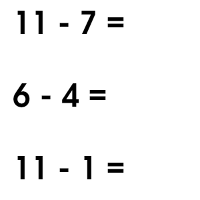### For kids to practice up to 10+10

By

Worksheets give kids practice, practice helps remember, and lets face it, at least some of math is memory work.

The worksheets available here (all free and easy to print, of course) each contain every addition and subtraction sum from 0+0 (or 0-0) up to 10+10 (or 20-10). Now that's a lot of sums. 11 pages of 22 each, in fact. Don't try to get your kid to complete the whole stack in 1 day! More like, 1 page a day would be reasonable.

If you want worksheets with more advanced addition problems, say for numbers with two or more digits, you need this addition worksheet generator.

I've provided ten worksheets. That's enough practice to keep your kids going for quite a long time!

• First, there are three addition worksheets, with 6 pages each. Download them here, here and here. Each of these has the 121 sums from 0+0 to 10+10, in a completely random order.
• Or if your child is learning subtraction, there are three subtraction worksheets, each with every sum from 0-0 to 20-10. Download them here, here and here.
• The next set of worksheets (download them here, here and here) have the addition and subtraction sums all mixed up together. That means they are 11 pages each, with 22 sums per page. Like I said, more than enough practice for most kids!• The last worksheet is special. Like the last three, it also has all the addition and subtraction sums mixed together. But the order is designed to help the kids learn some important concepts in addition and subtraction.

In the special worksheet, the sums are organised into groups of four, like you see in the picture on the right. Now the sums 3+8 and 8+3 are related to each other, and also to the sums 11-3 and 11-8. These relationships may be obvious to you and me, but a kindergarten kid has to learn them. Since the best way to learn something is to figure it out for yourself, I designed this worksheet to do just that.

As your child works through the worksheet (make sure he or she does the sums in order, column by column!) he or she will discover, not merely be taught, important concepts such as

• if a plus b is c, then so is b plus a!
• if a plus b is c, then c minus a is b! And vice-versa, too!!
• if c minus a is b, then c minus b is a!
Just remember to act surprised, and say "How clever you are!", when your precious angel shows you his or her latest discovery!